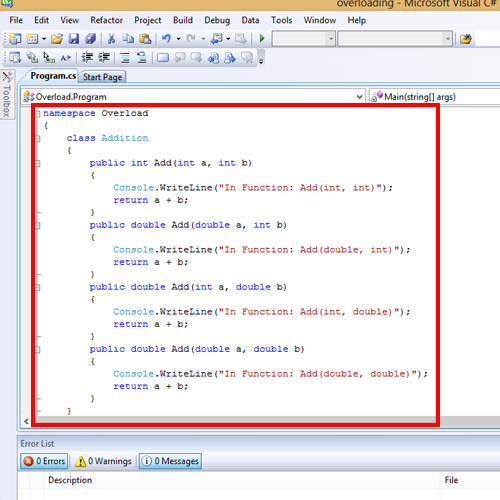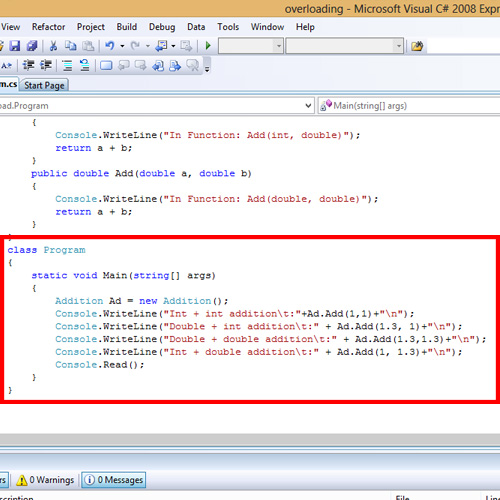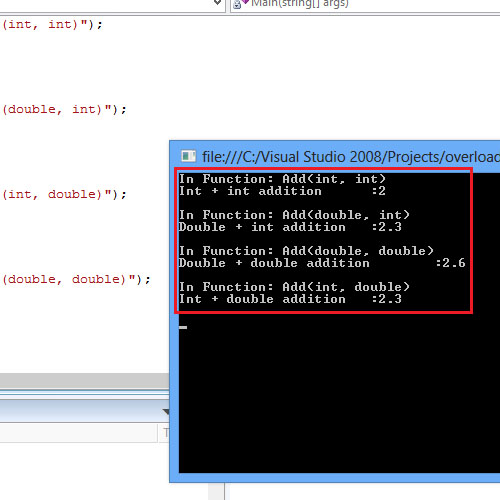Method overloading in C# is the most common way of implementing polymorphism. Method overloading can be achieved by defining two or more methods in a class with the same name. However each method should possess a different signature. Let’s consider an example method:
public void example(int a) { }
public void example(int a, int b) { }
public void example(string a) { }
public void example(int a, string b) { }
All the methods are of name example. They differ in the number of parameters passed to the function and the data type of the parameters. Thus method overloading can be a simple case of different data type to multiple parameters and optional parameters

Step 1: Implementation

The following code shows the use of method overloading in C#. In the code, the Add() method is overloaded. The Add() method is initialized four times and each time the signature of the method varies.
Depending on the parameters being passed, the respective method with the respective signature is called. It is the run-time decision of the compiler.Step 2: Calling the method

When the add() method is called from the main method, depending on the parameter data types, the respective add() method will be called. Thus the first method call has 2 integer parameters and this invokes the add() method that takes in 2 integer parameters. The following method calls the respective add methods().Step 3: Output

When the method is called in the Main method, the compiler finds the best fit, depending on the parameters of the function call. Thus, when Add(1, 1) is called, the compiler searches for a method that has the signature (int, int) respectively.Note that in overloading methods in C#, the signature of the method does not include the return type. Thus the compiler will not check for the return type of the methods and it may result in error.